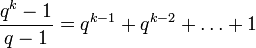# Equivalence of definitions of size of projective space

## Statement

### For a vector space over a prime field, or an elementary abelian group

Suppose$p$ is a prime number and$V$ is an elementary abelian group of order$p^k$, i.e., a vector space of dimension$k$ over the field of$p$ elements. Then, the following three sets have the same size:

1. The set of minimal subgroups of$V$, i.e., subgroups of order$p$.
2. The set of maximal subgroups of$V$, i.e., subgroups of order$p^{k-1}$.
3. The projective space for$V$ (this is a projective space of dimension$k - 1$ over the field of$p$ elements).

Moreover, the size of all three sets is:$\frac{p^k - 1}{p - 1} = p^{k-1} + p^{k-2} + \dots + 1$

### For a vector space over a finite field

Suppose$q$ is a prime power and$V$ is a vector space of dimension$k$ over the field with$q$ elements. Then, the following three sets have the same size:

1. The set of one-dimensional subspaces of$V$.
2. The set of codimension one subspaces of$V$.
3. The projective space for$V$ (this is a projective space of dimension$k - 1$ over the field of$q$ elements).

Moreover, the size of all three sets is:$\frac{q^k - 1}{q - 1} = q^{k-1} + q^{k-2} + \dots + 1$